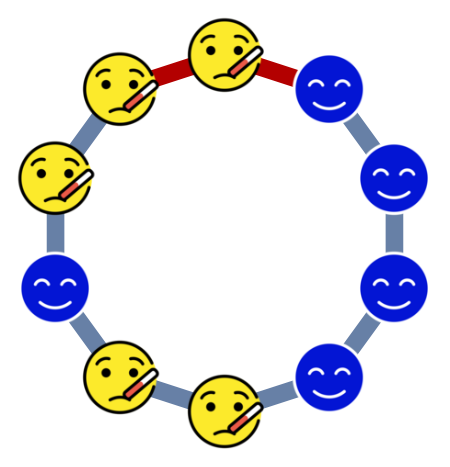# Bak-Sneppen model

I just did some simulation on Bak-Sneppen model, you can check it out at GitHub.

None

## Discrete Bak Sneppen model¶

In the discrete Bak-Sneppen model , each node on a circle of $n$ nodes is given a fitness of either $0$ or $1$. Then a node with fitness zero is chosen uniformly at random. This node together with its two neighbors are replaced by three nodes, each of which has a fitness $1$ with probability $p$ and $0$ with probability $1-p$.

If no one has fitness $0$, then the process restarts by choosing a uniform random node.

You can think of this model as if someone at work has Covid-19 😷️. So they and their colleagues sitting next to them have to go home and be replaced by people who (unfortunately) may or may not have Covid-19.

## An example¶

For example, suppose initially the cycle looks like this.Then the node at the top together with its two neighbors are selected.Three new nodes come to replace them, each with a random fitness. And the graph looks like this now.## A phase transition¶

We can view the status of the $n$ nodes on the circle as a Markov chain. This converges to a stationary distribution $\pi^{(n)}$ on $\{0,1\}^n$. Let $\nu^{(n)}(p)$ be the probability that the first node (actually any fixed node) on the circle has fitness $1$ in the stationary distribution.

It has been observed for a long time that if $p > 0.36$, then $\nu^{(n)} \to 1$. In other words, a fixed node eventually will have fitness $1$ almost all the time. Otherwise it will have fitness $0$ for some portion of time.

A recent paper by Stanislav Volkov  which proved the first part for $p > 0.411$.

For the second part an old paper  shows that it is true for $p<p_c$ where $p_c$ is non-zero constant. But no explicit bound on $p_c$ is given. So the problem now is if this gap can actually be closed? Or at least can we show that there is indeed a threshold?

## Bak-Sneppen on positive integers with two replacement¶

It seems a bit difficult to actually prove the threshold exists for the original model. So I come up with a simpler model that may be a slightly easier to analyze.

It works as follows

1. Put a label 1 😀️ on all positive integers $\ge 2$. Put label 0 😷️ on integer $1$.
2. Choose an integer $u$ with label 0 uniformly at random among all such integers.
3. Change the label of this integer to 1 with probability $p$, and keep it as $0$ with probability $1-p$.
4. Do the same for the for the integer $u+1$ (the neighbor on the right).
5. We say the process dies if there is no 0 integers left.

In other words, instead of replacing three people, we replace two. And the process runs on a line of infinite length instead of a cycle.

Here's my conjecture based on simulations.

1. If $p > 0.24$, then the process dies with probability $1$
2. If $p \in (0, 0.23)$, then the process has positive probability to run forever.

## Phase transition¶

In the simulation, if the process dies we just restart it.

We call the distance between the largest and the smallest integer with a label 0 the diameter of the process.

In :
ENV["GKS_ENCODING"]="utf8"
using Plots; using Pkg; Pkg.activate("..");
using Revise, BakSneppenSimulation

 Activating environment at ~/Dropbox/Research/2020/Bak-Sneppen/BakSneppenSimulation/Project.toml


### The threshold¶

For $p<0.23$, the picture looks like this. As you can see, the diameter and number of zeros all goes up forever.

In :
plotsim(simlinear(SimConfig(1000, 0.05, 2, 10^6)))

Out:

At $0.23$ the growth is not that smooth anymore.

In :
plotsim(simlinear(SimConfig(1000, 0.23, 2, 10^6)))

Out:

For $p=0.24$, it is clear that the process dies every time it starts

In :
plotsim(simlinear(SimConfig(1000, 0.24, 2, 10^6)))

Out:

### The percentage of zeros¶

Another interesting thing to notice is that the number of of zero divided by the diameter seems to be stable when $p$ is small.

My guess is that this ratio should be $1-2 p$. This is because if we assume that the positions of $0$ and $1$ are uniformly at random between the first and the last $0$'s (which they are not), then with $1-2 p$ percentage of $0$, the percentage of $0$ will remain stable in expectation.

Simulations seem to agree.

In :
plotzero(simlinear(SimConfig(1000, 0.05, 2, 10^6)))

Out:
In :
plotzero(simlinear(SimConfig(1000, 0.1, 2, 10^6)))

Out:
In :
plotzero(simlinear(SimConfig(1000, 0.15, 2, 10^6)))

Out:

## A generalization¶

More generally, we can play with parameters $l$ and $r$, which are the number of neighbors on the left/right which are replaced together with the chosen 0. So the model above corresponds to $l=0$ and $r=1$.

Simulation seems to suggest that as long as $r \ge 1$, there is always a threshold.

### $l=1$ and $r=1$¶

If we let $l=1$ and $r=1$, then not surprisingly we get the same threshold as $0.36$ as in the original circular model.

In :
plotsim(simlinear(SimConfig(1000, 0.36, 3, 10^6)))

Out:
In :
plotsim(simlinear(SimConfig(1000, 0.37, 3, 10^6)))

Out:

### $l=1$ and $r=2$¶

In this case, there seems to exist a threshold around $0.48$. That means the larger the $l$, the more likely for it to survive.

In :
plotsim(simlinear(SimConfig(1000, 0.48, 4, 10^6)))

Out:
In :
plotsim(simlinear(SimConfig(1000, 0.49, 4, 10^6)))

Out: